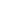028-87843859vip@cddingdang.com

# 酸、碱、强弱溶液与PH值的变化趋势以及溶解pH计算公式

[导读]：强酸、强碱溶液的ph值的变化趋势及的 ph计 算公式 (1)强酸溶液的 ph计 算，应用下式：[h+]溶=[h+]酸+[h+]水；由于[h+]水与[h+]酸相差倍数甚远，所以ph= -lg[h+]酸，即把水的电离忽略不计了。但溶液中[oh-]一定是由水电离来的，所以[oh-]溶=[oh-]水。 (2)如果强酸的 浓度 接近于10-7mol/l，那么[h+]溶=[h+]酸+[h+]水，水的电离不能忽略。ph= -lg[h+]溶====-lg (3)如果已知某多元强酸的物质的量的 浓度 (c)，那么：ph=

(1)强酸溶液的ph计算，应用下式：[h+]溶=[h+]酸+[h+]水；由于[h+]水与[h+]酸相差倍数甚远，所以ph= -lg[h+]酸，即把水的电离忽略不计了。但溶液中[oh-]一定是由水电离来的，所以[oh-]溶=[oh-]水。

(2)如果强酸的浓度接近于10-7mol/l，那么[h+]溶=[h+]酸+[h+]水，水的电离不能忽略。ph= -lg[h+]溶====-lg

(3)如果已知某多元强酸的物质的量的浓度(c)，那么：ph= -lg[h+]=-lg(n·c)　　(n为酸的元次)

(4)如果求强碱溶液的ph时， 应注意必须先求[oh-]或poh后，再求[h+]或ph，否则，易出错。[oh-]溶=[oh-]碱+[oh-]水；[h+]溶=[h+]水

(1)已知某一元弱酸的物质的量的浓度，则[h+]溶=[h+]酸+[h+]水；而水的电离能否忽略不计，应分析[h+]酸与[h+]水的倍 数。一般二者相差103或103以上，[h+]水可忽略不计。由于弱酸是部分电离，所以[h+]酸=α·c；[h+]溶=[h+]酸=α·c；ph= -lg[h+]= -lg(α·c)；也就是弱酸的ph计算与强酸的不同点，就是要利用电离度求[h+]。

(2)有关弱碱溶液的ph计算，首先要求poh或[oh-]，第二点要注意[oh-]应利用电离度。第三要考虑水的电离能否忽略不计。 ph=14-poh=14-lg[oh-]溶=14-lgα·c(当水的电离忽略时，弱碱为一元时)

## 酸、碱、强弱溶液与PH值的变化趋势以及溶解pH计算公式厂家优势特点Tag:

最新推荐信息